# How to Calculate End Value from CAGR in Excel (6 Methods)

Get FREE Advanced Excel Exercises with Solutions!

Compound Annual Growth Rate (CAGR) is a well-known term in financial sectors. The compound growth rate is calculated based on the investment. In this article, we will calculate the end value using CAGR in Excel with 6 easy methods.

## What is Compound Annual Growth Rate (CAGR)?

The compound annual growth rate is the rate of interest of investment comparing the end value and initial value with the number of years passed.

Mathematical Formula of CAGR:

CAGR=(EV/IV)^(1/n)-1*100

Where,

EV=End value

IV= Initial value

n= Number of Years

So, our desired end value,

EV=IV(CAGR/100+1)^n

We will calculate the CAGR first and then find the end value using that CAGR.

## 6 Methods to Calculate End Value from CAGR in Excel

We will show some methods to calculate the end value of CAGR in Excel.

The dataset below will be considered for calculation.

###We will calculate the CAGR from this dataset, then calculate the end value.

### 1. Use Simple Formula to Calculate End Value from CAGR

We will create a formula for CAGR calculation.

Now, add new cells for CAGR and End Value. We will calculate the end value for the year 2020 based on the previous data.

###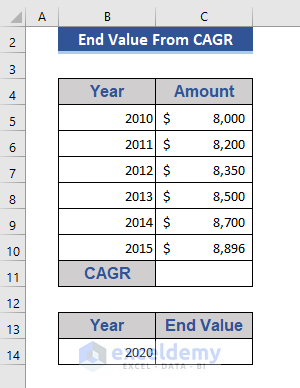Step 1:

• Go to Cell C11.
• Write the following formula.
`=(C10/C5)^(1/5)-1`

###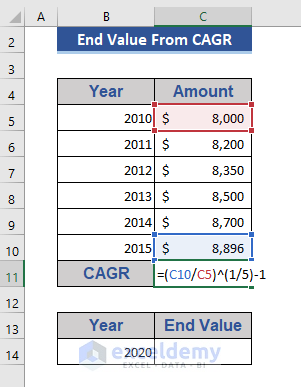Step 2:

• Now, press Enter.

###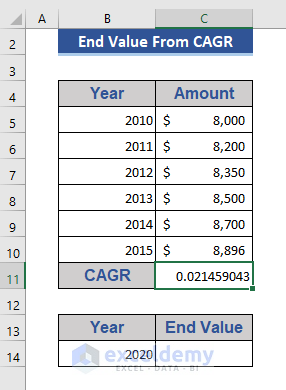Now, convert the growth rate into percentage form.

Step 3:

• Press Ctrl+1.
• Choose the Percentage option from the Number tab.
• Then press OK.

###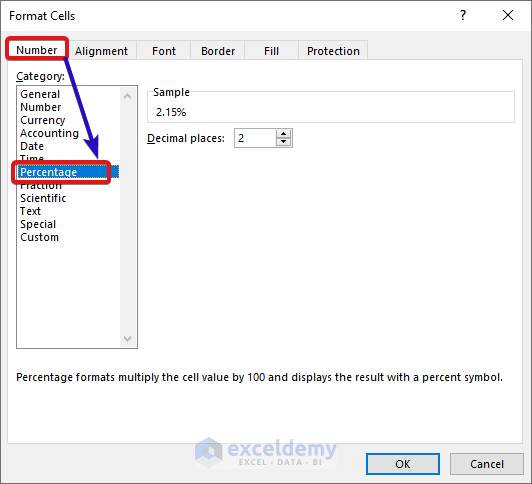Look at Cell C11 now.

###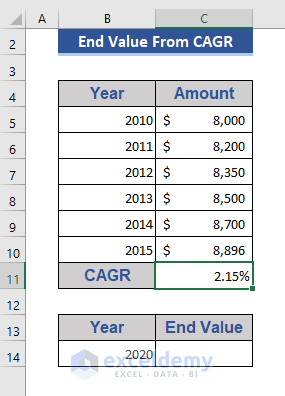The CAGR is presented in the proper form.

Step 4:

• Move to Cell C14.
• Input the formula below.
`=C5*((C11+1)^10)`

###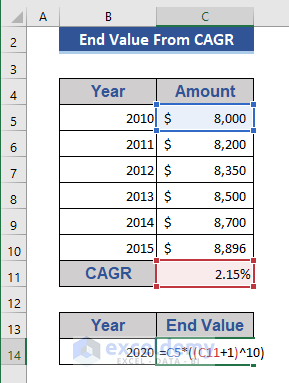Step 5:

• Now, press the Enter button.

###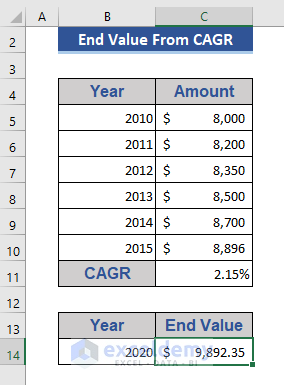Here, we get the end value for the year 2020 based on the previous values.

Read More: Excel Formula to Calculate Average Annual Compound Growth Rate

### 2. Excel RATE Function to Calculate CAGR and End Value

The RATE function is used to get the interest of investment annually.Step 1:

• Go to Cell C11.
• Put the formula below.
`=RATE(5,0,-C5,C10)`Step 2:

• Press the Enter button.Step 3:

• Now, we will calculate the end value on Cell C14.
• Copy and Paste the following formula.
`=C5*((C11+1)^10)`Step 4:

• Again, press the Enter button.We get the end value of the year 2020.

Read More: CAGR Formula in Excel: With Calculator and 7 Examples

### 3. Apply POWER Function to Determine the End Value from CAGR

The POWER function calculates the output of a number based on the given power.We will use this power function to calculate the end value of CAGR.

Step 1:

• Go to Cell C11.
• Write the formula below.
`=POWER(C10/C5,1/5)-1`Step 2:

• Click the Enter button.Step 3:

• Now, go to Cell C14 to calculate the end value.
• Put the formula on that cell.
`=C5*POWER(C11+1,10)`Step 4:

• Now, press the Enter button.Read More: Compound interest excel formula with regular deposits

### 4. Combine RRI and ROW Functions to Measure End Value

The RRI function provides an interest rate for the invested money.The ROW function is the reference of a row number.We will combine the RRI and ROW functions to get CAGR first.

Step 1:

• Write the formula based on the RRI and ROW function on Cell C11.
`=RRI(ROW(C10)-ROW(C5),C5,C10)`Step 2:

• Press the Enter button.Step 3:

• Go to Cell C14 and put the following formula.
`=C5*((C11+1)^10)`Step 4:

• Again, press the Enter button.Read More: Compound Interest Formula in Excel: Calculator with All Criteria

### 5. Use of GEOMEAN Function to Find the End Value from CAGR

The GEOMEAN function provides the geometric mean from a given range of data.The PRODUCT function returns the multiplication of multiple numbers.We add a column named Factor for this method.Step 1:

• Go to Cell C6. Put the following formula in that cell.
`=C6/C5`Step 2:

• Press Enter and pull the Fill Handle icon.Step 3:

• Go to Cell C11. Put the formula below.
`=GEOMEAN(D6:D10)-1`Step 4:

• Click the Enter button.Step 5:

• Now, go to Cell C14.
• Insert the formula based on the PRODUCT function.
`=PRODUCT(C5,((C11+1)^10))`Step 6:

• Finally, press the Enter button.Read More: How to Use Compound Interest Formula in Excel

### 6. Apply Excel FV Function to Calculate the End Value from CAGR

The FV function calculates the future value of any investment.We will calculate the future value from the dataset below.We will calculate the end value after 10 years of the initial investment with the given CAGR.

Step 1:

• Go to Cell C10.
• Write the following formula.
`=FV(C6,C7,,-C5,C9)`Step 2:

• Now, press Enter.Read More: How to Calculate Future Value When CAGR Is Known in Excel (2 Methods)

## Things to Remember

In the case of the FV and RATE function, the initial value must be negative in the formula.

## Conclusion

In this article, we’ve explained how to calculate the CAGR and the end value of the CAGR in Excel. I hope this will satisfy your needs. Please have a look at our website Exceldemy.com and give your suggestions in the comment box.

## Related ArticlesAlok Paul

Hello, this is Alok. I am working as an Excel & VBA Content Developer at Exceldemy. I want to provide solutions to various Excel-based problems. I completed my study at East West University major in Telecommunications Engineering. I love traveling, reading books, playing cricket.

We will be happy to hear your thoughtsAdvanced Excel Exercises with Solutions PDF